## Error message

• Deprecated function: The each() function is deprecated. This message will be suppressed on further calls in menu_set_active_trail() (line 2404 of /homepages/3/d408889334/htdocs/sg2/includes/menu.inc).
• Deprecated function: implode(): Passing glue string after array is deprecated. Swap the parameters in drupal_get_feeds() (line 394 of /homepages/3/d408889334/htdocs/sg2/includes/common.inc).

# 1.- Introduction

Simply stated, the Golden Ratio establishes that the small is to the large as the large is to the whole. This is usually applied to proportions between segments. This ratio has been venerated by every culture in the planet. We can find it in art, music composition, even in the proportions of our own body, and elsewhere in Nature "hidden" behind the Fibonacci sequence. In this site we also provide some examples of disciplines where the presence of the Golden Ratio was unsuspected until recently. Such is the case, for example, of Atomic Physics or DNA codon populations of the whole human genome.

In this article I will present the basics about the Golden Ratio. As I don't like to take things for granted, I don't expect you to do so, so I will try to provide short mathematical or geometrical demostrations of my statements (don't be afraid, you won't face any partial differential equation). We will be talking about the definition of the Golden Ratio, its geometrical construction, some mathematical properties, and some important geometrical objects where you can find it. The "applications" are left for the separate pages that you can find at the "And much more..." section, although I encourge you to read this article first.

# 2.- The Golden Ratio as a limiting value

It is important to stress that, although the Greeks gave to the Golden Ration its name Phi (φ), as its name indicates it is actually a ratio. This ratio can be obtained as a limiting form of the following general type of succession (we noticed this property after reading R.W. Ray's web page). Given two positive initial elements a0 and a1, compute a general element an by the addition of the two preceding ones:

$an+1=an+an-1$

As a consequence, the ratio of two consecutive elements in the series ${q}_{n}={a}_{n}/{a}_{\mathrm{n-1}}$ also satisfies a recurrence:

$an+1an=1+an-1an⇒qn+1=1+1qn$

If the consecutive ratios qn tend to a limiting value Q, this must satisfy the equation:

$Q=1+1Q$

This leads to the well known second order equation whose positive solution is φ:

$Q2=Q+1⇒Q>0Q=φ=5+12$

What this result states is that the ratio between consecutive values of any sequence of this type always aproaches φ. Notice that the Golden Ratio is connected with how the series is constructed, but not with any particular example of that construction. You could come to an infinite number of such sequences depending on the initial values a0 and a1. For example when a0=2 and a1=1 you get Lucas sequence:

$Lucas sequence:2,1,3,4,7,11,18,29,…$

 n L(n) qn = L(n)/L(n-1) 1 2 --- 2 1 1/2 = 0.500 3 3 3/1 = 3.000 4 4 4/3 = 1.333 5 7 7/4 = 1.750 6 11 11/7 = 1.571 7 18 18/11 = 1.636 8 29 29/18 = 1.611 9 47 47/29 = 1.620 10 76 76/47 = 1.617 Table 1: The quotient of consecutive elements in the Lucas sequence converge to the Golden Ratio.

But there is one very special sequence that is closely related to the Golden Ratio, and this is the Fibonacci sequence (a0=1, a1=1):

$Fibonacci sequence:1,1,2,3,5,8,13,21,34,55,…$

 n F(n) qn = F(n)/F(n-1) 1 1 --- 2 1 1/1 = 1.000 3 2 1/2 = 0.0500 4 3 3/2 = 1.500 5 5 5/3 = 1.666 6 8 8/5 = 1.600 7 13 13/8 = 1.625 8 21 21/13 = 1.615 9 34 34/21 = 1.619 10 55 55/34 = 1.617 Table 2: The quotient of consecutive elements in the Fibonacci sequence converge to the Golden Ratio.

Why is the Fibonacci sequence so special? Well, at least from the fact that, as Drunvalo Melchizedek points out in his first book "The Ancient Secret of the Flower of Life", Nature uses this property to construct sequences of lengths that converge to the Golden Ratio, such as in the distances between successive branches of a tree, or successive leaves in a branch, or the dimensions of our own body: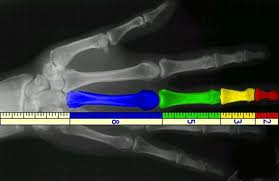Figure 1: Two examples of biological constructs that obey the Fibonacci recurrence.

The Fibonacci sequence has many more interesting properties and is more involved in the reality that we perceive than we suspect. We are preparing an article on this subject that will be available soon in the corresponding section of our site.

Before going on, we should point out some mathematical properties that follow from the fact that φ satisfies the second order equation φ2 = φ + 1:

 Index Power Inverse 1 φ 1/φ = φ - 1 2 φ2 = φ + 1 1/φ2 = 1 - 1/φ = 2 - φ 3 φ3 = φ2 + φ = 2φ + 1 1/φ3 = 1/φ-1/φ2 = 2φ - 3 4 φ4 = φ3 + φ2 = 3φ + 2 1/φ4 = 1/φ2-1/φ3 = 5 - 3φ 5 φ5 = φ4 + φ3 = 5φ + 3 1/φ5 = 1/φ3-1/φ4 = 5φ-8 ... ... ... n φn = φn-1 + φn-2 = F(n)φ + F(n-1) 1/φn = 1/φn-2-1/φn-1 = (-1)n·[F(n+1) - F(n)φ] Table 3: Powers of the Golden Ratio

where F(n) is the nth element of the Fibonacci sequence starting as F(0) = F(1) = 1.

By the way, it is inmediately apparent from Table 3 that φ itself establishes a "Fibonacci" sequence, which at the same time is a geometric progression, both above and below unity:

$…,1φ3,1φ2,1φ,1,φ,φ2,φ3,…$

# 3.- The Golden Ratio as a mean value

The Golden Ratio is also a way of dividing a segment into two pieces that reproduce the statement at the beginning of this article: the small is to the large as the large is to the whole. Consider the following segment:Its division into Golden Ratio can be expressed mathematically as follows:

$ab=a+ba$

This relationship can be treated in terms of the quotient Q=a/b, which leads to the same equation as before:

$ab=1+ba⇒Q=1+1Q⇒Q=ab=φ=5+12$

From this we deduce that each part (the big part a or the small part b) have the following proportions with respect to the total segment (a+b):

$ba+b=11+ab=11+φ=1φ2$

$aa+b=ab1+ab=φ1+φ=φφ2=1φ$

In the special case of a unit segment, the Golden Ratio provides the only way to divide unity in two parts that are in a geometric progression:Figure 2: The Golden Ratio is the only way of dividing unity it in two parts that are in geometric progression.

Before we go into the geometric construction methods, we would like to stress another fact: the division of a segment in Golden Ratio is a process that can be iterated indefinitely, and the first division provides all the necessary elements to do it. The following figure illustrates the process of iterative division into successive Golden ratios. This process can also be iterated "outwards" using increasing powers of φ (see Table 3):Figure 3: Iterative division of a segment into Golden ratios.

# 4.- Geometric construction methods

There are many ways in which a segment can be geometrically divided according to the Golden Ratio. All of them need only a ruler and a compass (no scientific calculator!!). We are going to show three of them. In the first one (Method A, Figure 4) you simply need to compute the midpoint of a segment twice: starting with segment AA', compute its midpoint M. Then mark point B such that segment MB has the same length as AA', and calculate its midpoint M'. Finally, mark point C such that M'C=MB and that's all. You end up with a segment AC that is divided into Golden segments AB and BC. That is to say:

$ABBC=ACAB=φ$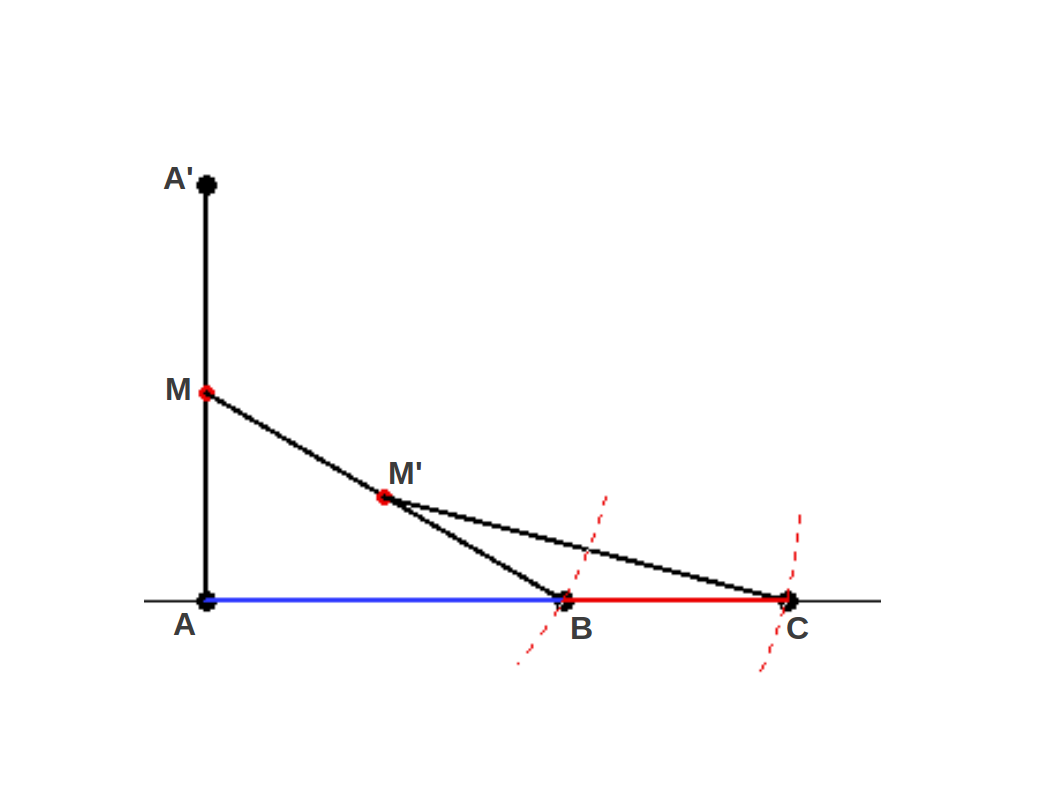Figure 4: METHOD A: Geometric construction of the Golden Ratio using two midpoints. With this method, you don't know a priori the segment size AC.

The other two methods have in common the usage of a 1-1/2 triangle (that is, a right angle triangle with one side half the other long). In method B you start from segment AC and compute its midpoint M. Then you rise half the length of the segment, MC, perpendicularly to get point C' and the 1-1/2 triangle ACC' (whose diagonal is $\sqrt{5}\mathrm{/2}$). Then you take the hight of the triangle onto the hypothenuse AC' to get point B', and finally you take length AB' down to get point B, which divides the original segment AC into Golden Ratio, so that you have again:

$ABBC=ACAB=φ$Figure 5: METHOD B: Geometric division of a segment in Golden Ratio using two arcs. The original segment gets divided in two golden parts.

The final method (method C) starts from a square of side length AB (Figure 6). You compute the midpoint of side AB and throw a circle putting the compass at this point and joining points D and C. And that's it: segment AC is divided into Golden ratios at point B, so that again

$ABBC=ACAB=φ$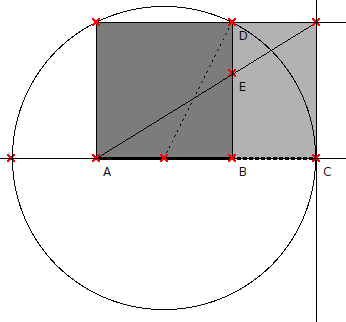Figure 6: METHOD C: Geometric determination of a segment AC such that AB and BC are in Golden Ratio. The process produces a Golden Rectangle, that contains a square and a smaller Golden Rectangle inside.

The third method has the advantage that you end up with a Golden Rectangle, that is, a rectangle whose sides are related by the Golden Ratio. And this process can be iterated indefinitely without using any further circle, just drawing the diagonals of each rectangle. For example the small golden rectangle in Fig.6 (light grey) contains an even smaller rectangle inside determined by point E, which divides side BD into Golden parts BE and ED (the reader should notice that in a general rectangle the lengths BE and BC will not necessarily be equal, whereas in a Golden Rectangle they are). Therefore, each new Golden Rectangle in this iterated process is composed of a square and a smaller Golden Rectangle. This construction process allows to build the Golden Spiral:Figure 7: The Golden Spiral

Although the three geometrical methods of constructing φ are equivalent, each one has its preferred usage. For example, method B is used when one wants to divide a segment of known length into two subsegments that obey the Golden Ratio. On the other hand, method C starts from a segment that will end up being the long part of the Golden Ratio division of the final segment AC. Method A can be used when you don't have any segment that you want to divide and you simply need the ratio. Then you can translate this ratio to any desired segment by similarity.

# 5.- Golden Triangle and Golden Gnomon

The Golden Ratio arises in many geometric constructions. One of them is the Golden Triangle. It is an isosceles triangle whose lateral sides are in Golden proportion to the base, which is usually taken as unity (Figure 8). What's the value of the angle θ? You can calculate it, but we're going to deduce it in the next figure.Figure 8: The Golden Triangle

When you swing an arc from the base of this triangle upwards, another isosceles triangle apears inside. This construction is usually called the Golden Gnomon. Here we show it before determining the values of the different angles and the side size L (Figure 9).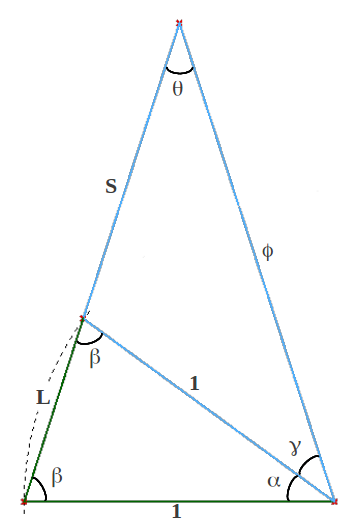Figure 9: The Golden Gnomon (preliminary)

From the fact that the big outer and the small inner triangles are both isosceles and share two equal angles β, it follows that α = β. That is, the inner and the outer triangles are similar because they have the same angles. That means that the base of the inner triangle obeys this similarity:

$φ1=1L⇒L=1φ$

Therefore

$S=φ-L=φ-1φ=1$

from which the upper blue triangle is also isosceles. This implies that γ = θ, so that actually the blue segment with length 1 bisects the angle β of the right. This property also allows us to determine the value of the angles β and θ in the Golden Triangle:

$α=γ=θ⇒θ=β2⇒2β+β2=180⇒β=25180=72⇒θ=36$

This leads to the picture of the Golden Gnomon that you can find elsewhere:Figure 10: The Golden Gnomon

# 6.- The Golden Ratio in the pentagon

The pentagon is the polygon that naturally contains the Golden Ratio. Quoting Robert Lawlor  "the pentagon [is] the symbol of life, with its fivefold
symmetry which appears only in living organisms"
. The pentagon could actually be defined as the regular polygon of five sides whose diagonals are in a Golden Ratio with its sides. This provides a direct method of building a pentagon as we show in the following picture:Figure 11: The construction of the pentagon from the Golden Ratio.

Starting with a side AB, you compute points C and C' which extend this segment in Golden Ratio (CB/AB = AC'/AB = φ). Then the length BC becomes the diagonal of the pentagon (for example BD and BD' in the figure) and allows you to locate its vertices.

The Golden Triangle and the Golden Gnomon are naturally contained in the pentagon (Figure 12). This demostrates in a different way that in the pentagon the ratio of the diagonal to the side is φ.(a) Golden Triangle in the pentagon (b) Golden Gnomon in the pentagon Figure 12

When you draw all the diagonals in the pentagon you end up with the pentagram. The pentagram shows that the Golden Gnomon, and therefore Golden Ratio, are iteratively contained inside the pentagon (Figure 13).(a) Iterated Golden Gnomons in the pentagon. (b) Successive Golden Ratio proportions in the pentagram Figure 13

I would like to end this introduction to the Golden Ratio showing a geometric construction that hides many proportions of Sacred Geometry. It is the squaring of the circle analised in  (Figure 14).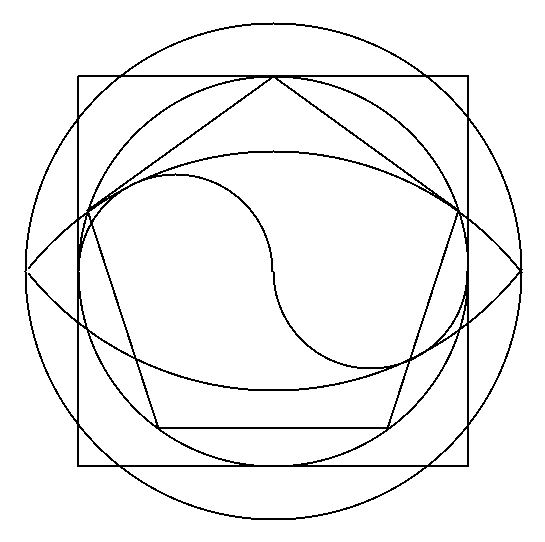Figure 14: The squaring of the circle.

We leave as an exercise to the reader to calculate the Golden proportions contained in this construction (Figure 15). I would like to stress that the outer square and the outer circle have almost the same area, and this is related with a long known simple relationship between two master generative numbers: φ and π.

$4π≈φ$Figure 15: Golden proportions in the squaring of the circle

# 7. References

Lawlor, Robert: "Sacred Geometry. Philosophy and Practice", Thames and Hudson, 1982, ISBN 0-500-81030-3.